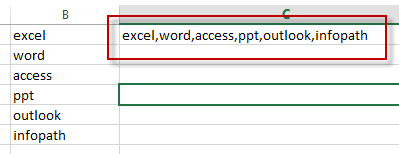# Convert Column to Comma Separated List in Excel

This post will guide you how to convert column list into a comma separated list in one cell in Excel. How do I convert a column into a comma separated list with a formula in Excel. Or how to convert a column into comma delimited list in Excel.

## Convert Column to Comma Separated List in Excel

Assuming that you have a list of data in range B1:B6, and you want to convert all values in column B to comma separated list in another cell. How to achieve it.

You can create a formula based on the TEXTJOIN function to achieve the result. Just like this:

`=TEXTJOIN(“,”,TRUE,B1:B6)`

Type this formula into a blank cell and press Enter key in your keyboard.

You can also use an Excel VBA macro to quickly convert column to a comma separated list in Excel. Just do the following steps:

#1 open your excel workbook and then click on “Visual Basic” command under DEVELOPER Tab, or just press “ALT+F11” shortcut.#2 then the “Visual Basic Editor” window will appear.

#3 click “Insert” ->”Module” to create a new module.#4 paste the below VBA code into the code window. Then clicking “Save” button.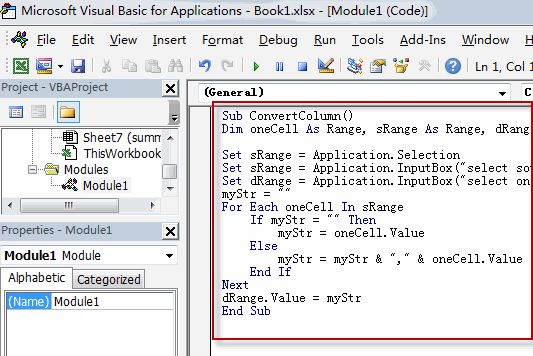```Sub ConvertColumn()
Dim oneCell As Range, sRange As Range, dRange As Range
myStr = ""

Set sRange = Application.Selection
Set sRange = Application.InputBox("select source Range:", "ConvertColumn", sRange.Address, Type:=8)
Set dRange = Application.InputBox("select one single cell as destination cell:", "ConvertColumn", Type:=8)

For Each oneCell In sRange
If myStr = "" Then
myStr = oneCell.Value
Else
myStr = myStr & "," & oneCell.Value
End If
Next
dRange.Value = myStr
End Sub```

#5 back to the current worksheet, then run the above excel macro. Click Run button.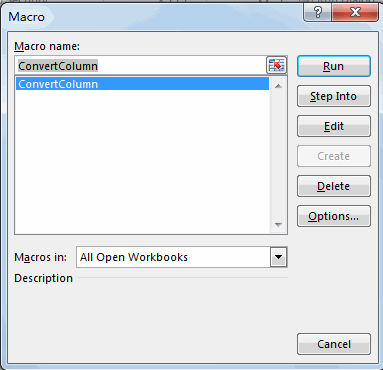#6 select the source range, such as: B1:B6.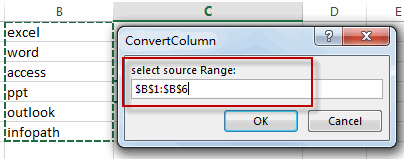#7 select one blank cell to place the list that is separated by comma. click Ok button.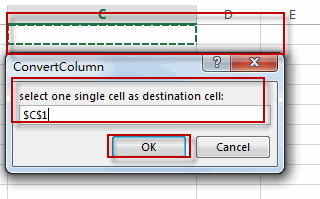#8 lets see the result.